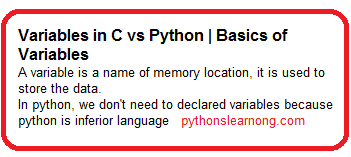Variables in C vs Python | Basics of Variables

## Variables in C vs Python | Basics of Variables

### Basics of Variables in Python

• A variable is a name of memory location, it is used to store the data.
• In python, we don’t need to declared variables because python is inferior language

### Object Identity in python

``````a=50
b=a
print(id(a))
print(id(b))
# Resigned variables a
a=500
print(id(a))``````

In the above program we declared two variables i.e. a and b. python is inferior language so it’s not need to declared variables as int types.

``````1407349
1407349
2456783``````

Above output windows show you addresses for a and b variables is same bur after resigned the variable addresses of a is change.

### Basics of variables in c

• Similar like python in c variables is a name of memory location it is used to store the data.
• Syntax of variables in c : type variables list;
• for example int a, float b, char c.

### Types of variables in c

1. Local Variables
2. Global variables
3. static variables
4. Automatic variables
5. Extern variable

### 1. Local Variables

A variable that is declared between the function in program is called a local variable.

It must be declared at the start of the function.

``````For exam:
void main()
{ int a=15;
}``````

#### 2. Global Variable

A variable that is declared outside the main function is called a global variable.
Any function can change the value of the global variable.

``````int value=20;//global variable
void function(){
int x=10;//local variable
} ``````

#### 3. Static Variable

A variable that is declared with the static keyword is called static variable.

``````void function(){
int x=10;//local variable
static int y=10;//static variable
x=x+1;
y=y+1;
printf("%d,%d",x,y);
}``````

#### 4. Automatic variable

Automatic variable are similar like local variable. they are define with auto keywords

``````void main()
{int a=10;  //local variable
auto int a=15; //Automatic variable
}``````

#### 5. Extern Variable

We know that we can share a value of variable in multiple source files by using an external variable or keywords.
Similar Auto variable, to declare an external variable, you need to use extern keyword.

``extern int z=10;//external variable``

#### Object Identity in C

``````#include<stdio.h>
int a;
int b;
int main()
{
a=50;
b=a;
printf("%d\n",a);
printf("%d\n",b);
a=100;
printf("%d",a);
return 0;
}``````

We include header file first then declared variables like int (integer) and write the code in main function.

``````1567945
1234325
1567945``````

Summary:

In this article we saw basics of variables in c and python so in the next article we will see Local and global variable.

### sachin Pagar

I am Mr. Sachin pagar Embedded software engineer, the founder of Sach Educational Support(Pythonslearning), a Passionate Educational Blogger and Author, who love to share the informative content on educational resources.